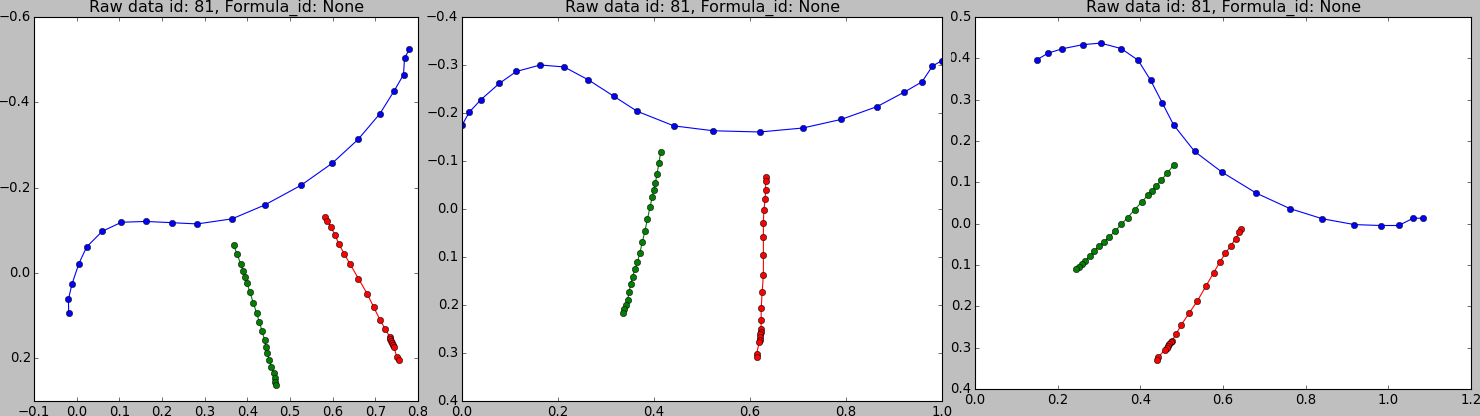# Data Multiplication¶

The automatic data multiplication works like this:

for algorithm in multiplication_queue:
new_training_set = []
for recording in training_set:
samples = algorithm(recording)
for sample in samples:
new_training_set.append(sample)
training_set = new_training_set
return new_training_set


Warning

The create_ffile procedure replaces the current set of recordings by the set returned by the data multiplication steps.Data multiplication algorithms.

Each algorithm works on the HandwrittenData class. They have to be applied like this:

>>> import data_multiplication as multiply
>>> a = HandwrittenData(...)
>>> multiplication_queue = [multiply.copy(10),
mulitply.rotate(-30, 30, 5)
]
>>> x = a.multiply(multiplication_queue)

class hwrt.data_multiplication.Multiply(nr=1)

Copy the data n times.

class hwrt.data_multiplication.Rotate(minimum=-30.0, maximum=30.0, num=5)

Add rotational variants of the recording.

hwrt.data_multiplication.get_data_multiplication_queue(model_description_multiply)

Get features from a list of dictionaries

>>> l = [{'Multiply': [{'nr': 1}]},              {'Rotate': [{'minimum':-30}, {'maximum': 30}, {'step': 5}]}]
>>> get_data_multiplication_queue(l)
[Multiply (1 times), Rotate (-30.00, 30.00, 5.00)]


Preprocessing

Features